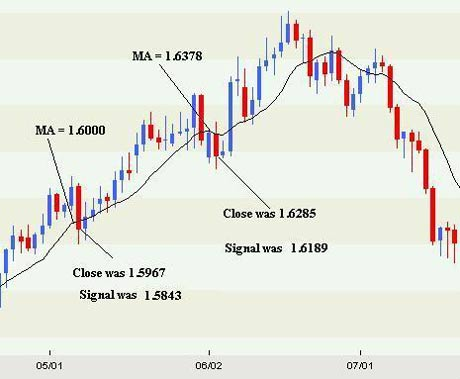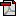Home | Previous | Next Lesson

This Lesson Can Be Printed See Instructions Below

# Range Signal Filter

In this lesson I am going to disclose a really useful technique to use with moving averages.

 In this lesson I am going to disclose a really useful technique to use with moving averages. Although I am not a big fan of moving averages as a sole method of trading: if used correctly with another indictor you can greatly increase the accuracy of their use. The main problem as I am sure you are aware by now is, if you use any kind of moving average as a signal generator the whipsaw kill's you. The secret of course is how do you use a moving average and not get whipsawed as frequently? I have found that if I use a filter with the moving average to help identify a true break; it keeps you in the trade longer and stops you entering on false break.

I have constructed my own little filter which, I find very useful. I first take note of the open, high, low and close of the time period I am following. Although I take note of the open and close we wont use them in our calculations.

Next I calculate the difference between the high and the low (range) of that period. From there we can make a simple 3 period moving average of the last 3 range calculations. The last part is to subtract the 3 period average of the ranges from the moving average we are using - in this case a 10 period moving average.

So how do we use this information? Well, let's assume that you are using a moving average: any moving average e.g. a 10 period moving average of the closing price.

Normally a signal would be generated if the closing price were either above or below the moving average. By adding a little filter of the 3 period average of the ranges we can better determine whether the moving average has in fact been breached.

Have a look at the table below.First column Date Second column Open Third column High Forth column Low Fifth column Close Sixth column Difference between the high and low Seventh column The 3 day average of the range (High - Low) Eighth column An average of the last 10 closing prices. Ninth column Signal - the10 period MA minus the 3 period MA of the ranges.As you can see from the chart above of the British Pound versus the Us Dollar: on the 7th May the close of the daily bar was 1.5967. The 10-day moving average of the closing price was 1.6000. A close below the average would normally indicate that you should either close or tighten your position. If we apply the 3-day filter of the ranges then the signal would actually be a close below 1.5843, which it closed above.

The next occurrence of closes below the moving average happened on the 2nd, 3rd and 4th of June. Of the 3 days, the only day that would have been of concern would have been the 3rd June. On that day the Moving Average was 1.6378 and the close of the day was 1.6285. After we applied the range signal of 1.6189 it is simple to see that we were in no danger of a reversal on that day.

Don't complicate this. The example above is for a long trade. All you are doing is subtracting the average of the last 3 ranges from the 10 period moving average (if that is what you are using). Once there is a close below that number the trend is assumed to have changed to down. On the short side, all you are doing is adding the average of the last 3 ranges to the 10 period average (if that is what you are using) and if the close is above that number then the trend is assumed to have changed to long.

You can experiment with the different moving averages and the average of the ranges looked at. The point is, if you are applying basic technical analysis such as trend lines or indicators: you can use this range average as a confirming signal.

You can also build it into an existing method to help improve results. You might also want to look at constructing a system based on this filter.

Good Trading

Best Regards
Mark McRae

Information, charts or examples contained in this lesson are for illustration and educational purposes only. It should not be considered as advice or a recommendation to buy or sell any security or financial instrument. We do not and cannot offer investment advice. For further information please read our disclaimer.To PRINT or save a copy of this lesson in PDF format simply click the PRINT link. This will open the lesson in a PDF format which, you can then PRINT. If you are unfamiliar with PDF or don't have a FREE copy of Arobat Reader see instructions.